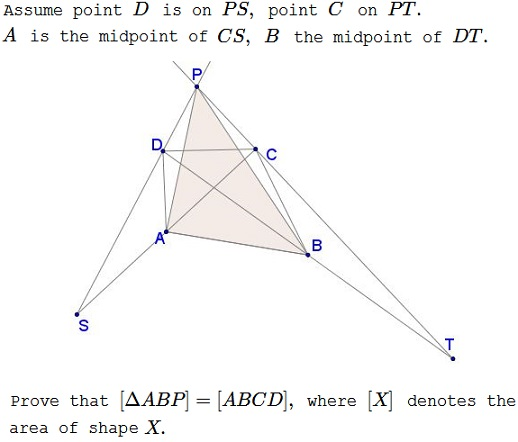# A Problem from Greece via Peru

### Source### Problem### Solution 1

The problem is of affine character: affine transformation preserve the ratio of parallel segments and, therefore, that of areas of any two shapes. We thus can specify the coordinates of any three of the given points arbitrarily. WLOG, let's choose $S=(-1,0),\,$ $T=(1,0),\,$ $P=(0,1).\,$ $SP\,$ is then described by $y=x+1\,$ so that we can choose point $D=(s,s+1)\,$ for some $s.\,$ Similarly, $C=(t,1-t)\,$ for some $t.\,$ Further $\displaystyle A=\frac{C+S}{2}=\left(\frac{t-1}{2},\frac{1-t}{2}\right)\,$ and $\displaystyle B=\frac{D+T}{2}=\left(\frac{s+1}{2},\frac{s+1}{2}\right).$

Now,

\displaystyle \begin{align} 2[\Delta ABP] &= \left|\begin{array}{ccc}\,\frac{t-1}{2}&\frac{1-t}{2}&1\\\frac{s+1}{2}&\frac{s+1}{2}&1\\0&1&1\end{array}\right|\\ &=\frac{1}{4}[(t-1)(s+1)-(1-t)(s+1)]-\frac{1}{2}[(s+1)-(t-1)]\\ &=\frac{1}{2}(st+1). \end{align}

Thus, $\displaystyle [\Delta ABP]=\frac{1}{4}(st+1).\,$

On the other hand, $\displaystyle \overrightarrow{BD}=\left(\frac{s-1}{2},\frac{s+1}{2}\right)\,$ and $\displaystyle \overrightarrow{AC}=\left(\frac{t+1}{2},\frac{1-t}{2}\right).\,$ So, using the vector product,

\displaystyle \begin{align} 2[ABCD] &= |\overrightarrow{BD}\times\overrightarrow{AC}|\\ &=\left|\begin{array}{cc}\frac{t+1}{2}&\frac{1-t}{2}\\\frac{s-1}{2}&\frac{s+1}{2}\end{array}\right|\\ &=\frac{1}{4}[(t+1)(s+1)-(1-t)(s-1)]\\ &=\frac{1}{2}(st+1). \end{align}

It follows that $\displaystyle [ABCD]=\frac{1}{4}(st+1)=[\Delta ABP].$

### Solution 2

Let $E\,$ be the midpoint of $CD.\,$ Then $BE\,$ is a midline in $\Delta CDT\,$ such that $BE\parallel PT.\,$ Similarly, $AE\parallel PS.$If so, $BCPE\,$ is a trapezoid and so is $AEPD.\,$ Let $F\,$ be the point of intersection of the diagonals of $BCPE,\,$ and $G\,$ that of $AEPD.\,$ We know that $[\Delta BCF]=[\Delta EFP]\,$ and $[\Delta AGD]=[\Delta GEP].\,$ It follows that

\displaystyle \begin{align} [ABCD]&=[\Delta AGD]+[ABFG]+[\Delta BCF]\\ &=[\Delta GEP]+[ABFG]+[\Delta EFP]\\ &=[\Delta ABP]. \end{align}

### Acknowledgment

This problem has been posted by Thanos Kalogerakis (Greece) at the Peru Geometrico facebook group and at the CutTheKnotMath facebook page. Solution 2 is by Ismail Erciyes (Turkey).